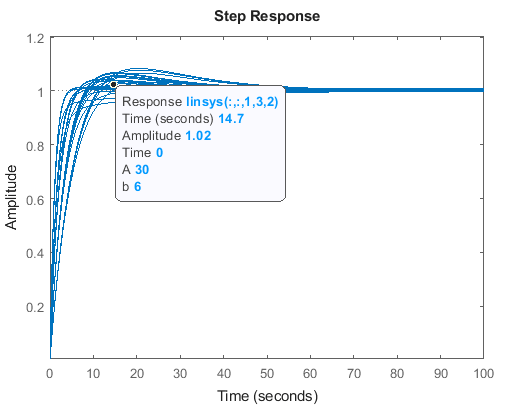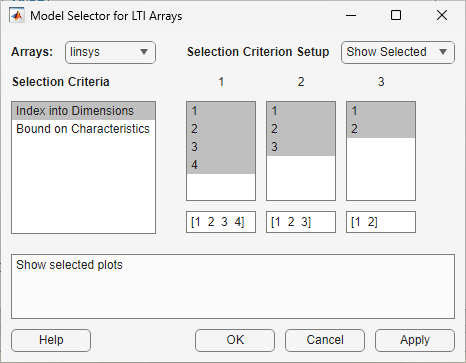# Analyze Command-Line Batch Linearization Results Using Response Plots

This example shows how to plot and analyze the step response for batch linearization results obtained at the command line. The term batch linearization results refers to the `ss` model array returned by the `slLinearizer` interface or `linearize` function. This array contains linearizations for varying parameter values, operating points, or both, such as illustrated in Batch Linearize Model for Parameter Variations at Single Operating Point and Vary Operating Points and Obtain Multiple Transfer Functions Using slLinearizer Interface. You can use the techniques illustrated in this example to analyze the frequency response, stability, or sensitivity for batch linearization results.

### Batch Linearize Model

For this example, batch linearize the `watertank` Simulink® model. The following code linearize the model for four simulation snapshot times, `t = [0 1 2 3]`. At each snapshot time, the model parameters, `A` and `b`, are varied. The sample values for `A` are `[10 20 30]`, and the sample values for `b` are `[4 6]`. The `slLinearizer` interface includes analysis points at the reference signal and plant output.

Open the model.

`mdl = "watertank"`
```mdl = "watertank" ```
`load_system(mdl)`

Create an `slLinearizer` interface for the model.

```sllin = slLinearizer(mdl,... [mdl + "/Desired Water Level",mdl + "/Water-Tank System"]);```

Specify the parameter grid.

```[A_grid,b_grid] = ndgrid([10,20,30],[4 6]); params(1).Name = 'A'; params(1).Value = A_grid; params(2).Name = 'b'; params(2).Value = b_grid;```

Set the parameters in the `slLinearizer` interface.

`sllin.Parameters = params;`

Set the operating point snapshot times in the `slLinearizer` interface.

`sllin.OperatingPoints = [0,1,2,3];`

Obtain the linearized model from the reference signal to the plant output.

```linsys = getIOTransfer(sllin,... "Desired Water Level","Water-Tank System");```

### Plot Step Responses of the Linearized Models

To plot the step responses of the linearized models, use the `stepplot` function.

`stepplot(linsys)`The step plot shows the responses of every model in the array. This plot shows the range of step responses of the system in the operating ranges covered by the parameter grid and snapshot times.

To view the parameters associated with a particular response, click the response on the plot.A data tip appears on the plot, providing information about the selected response and the related model. The last lines of the data tip show the parameter combination and simulation snapshot time that yielded this response. For example, in this previous plot, the selected response corresponds to the model obtained by setting `A` to `30` and `b` to `4`. The software linearized the model after simulating the model for three time units.

### View Step Response for Subset of Results

Suppose you want to view the responses for models linearized at a specific simulation snapshot time, such as two time units. Right-click the plot and select Array Selector. The Model Selector for LTI Arrays dialog box opens.The Selection Criterion Setup panel contains three columns, one for each model array dimension of `linsys`. The first column corresponds to the simulation snapshot time. The third entry of this column corresponds to the simulation snapshot time of two time units, because the snapshot time array was `[0,1,2,3]`. Select only this entry in the first column.Click OK. The plot displays the responses for only the models linearized at two time units.### Plot Step Response for Specific Parameter Combination and Snapshot Time

Suppose you want to examine only the step response for the model obtained by linearizing the `watertank` model at `t = 3`, for `A = 10` and `b = 4`. To do so, you can use the `SamplingGrid` property of `linsys`, which is specified as a structure. When you perform batch linearization, the software populates `SamplingGrid` with information regarding the variable values used to obtain the model. The variable values include each parameter that you vary and the simulation snapshot times at which you linearize the model. For example:

`linsys(:,:,1).SamplingGrid`
```ans = struct with fields: Time: 0 A: 10 b: 4 ```

Here, `linsys(:,:,1)` refers to the first model in `linsys`. This model was obtained at simulation time `t = 0`, for `A = 10` and `b = 4`.

Use array indexing to extract from `linsys` the model obtained by linearizing the watertank model at `t = 3`, for `A = 10` and `b = 4`.

```sg = linsys.SamplingGrid; sys = linsys(:,:,... sg.A == 10 & sg.b == 4 & sg.Time == 3);```

The structure `sg` contains the sampling grid for all the models in `linsys`. The expression `sg.A == 10 & sg.b == 4 & sg.Time == 3` returns a logical array. Each entry of this array contains the logical evaluation of the expression for corresponding entries in `sg.A`, `sg.B`, and `sg.Time`. `sys` is a model array that contains all the `linsys` models that satisfy the expression.

View the step response for `sys`.

`stepplot(sys)`